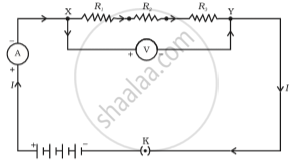SSC (Marathi Semi-English) 10thMaharashtra State Board
Share

# Resistance of a System of Resistors - Resistors in Series

#### description

• Series combination of resistors

#### notes

RESISTANCE OF A SYSTEM OF RESISTORS:-

There are two methods of joining the resistors together: series & parallel.

Resistors in Series:The total resistance of the system is equal to the sum of the resistance of all the resistors in the system.

If the total resistance is R

Resistance = R1,R2,R3,......Rn

Therefore R=R1+R2+R3+.......+Rn

In series the Voltage (V) varies and  current (I) remains the same.

S